Courses

NCERT Solution (EX - 2.3) - Chapter 2: Linear Equations in one variable, Maths, Class 8 Notes - Class 8

Class 8: NCERT Solution (EX - 2.3) - Chapter 2: Linear Equations in one variable, Maths, Class 8 Notes - Class 8

The document NCERT Solution (EX - 2.3) - Chapter 2: Linear Equations in one variable, Maths, Class 8 Notes - Class 8 is a part of Class 8 category.
All you need of Class 8 at this link: Class 8

Linear Equations in one variable

Exercise 2.3

Question 1:
Solve the following equations and check your results: 3x = 2x +18

3x = 2x +18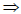3x - 2x = 18x = 18
To check:
3x = 2x -183x18 =  2x18+1854 = 36+1854 = 54L.H.S. = R.H.S.
Hence, it is correct.

Question 2:
Solve the following equations and check your results: 5t - 3 = 3t - 5

5t -3 = 3t - 55t -3t = 5+32t =- 2t= -2/2 = -1

To check:
5t 3  3t 55x(-1)- 3 = 3x(-1)- 5- 5 - 3 = - 3- 5- 8 = -8L.H.S. = R.H.S.
Hence, it is correct.

Question 3:
Solve the following equations and check your results: 5x 9  53x

5x + 9 = 5+3x5x - 3x = 5- 92x = -4
To check:
5x 9  53x5x(-2)+ 9 = 5 + 3x(-2)-10+9 = 5- 6-1= -1L.H.S. = R.H.S.
Hence, it is correct.

Question 4:
Solve the following equations and check your results: 4z +3 = 6 + 2z

4z+3=6+2z4z-2z=6-32z=3z = 3/2

To check:
4z +3 = 6 + 2z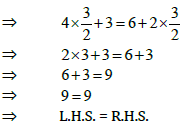Hence, it is correct.

Question 5:
Solve the following equations and check your results: 2x -1=14- x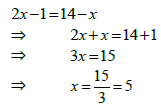To check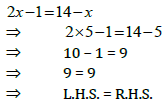Hence, it is correct.

Question 6:
Solve the following equations and check your results: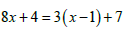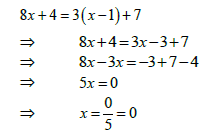To check: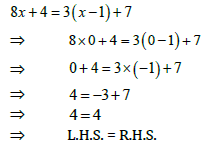Hence, it is correct.

Question 7:
Solve the following equations and check your results: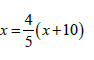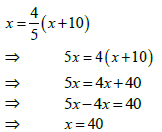To check: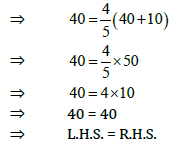Hence, it is correct.

Question 8:
Solve the following equations and check your results: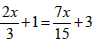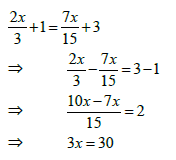x=30/3 =10

To check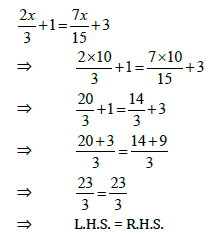Hence, it is correct.

Question 9:
Solve the following equations and check your results: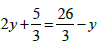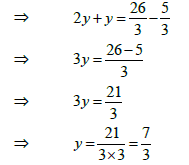To check: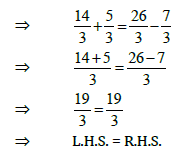Hence, it is correct.

Question 10:
Solve the following equations and check your results: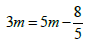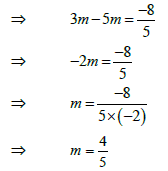To check: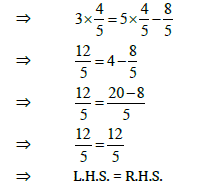Hence, it is correct.

The document NCERT Solution (EX - 2.3) - Chapter 2: Linear Equations in one variable, Maths, Class 8 Notes - Class 8 is a part of Class 8 category.
All you need of Class 8 at this link: Class 8Use Code STAYHOME200 and get INR 200 additional OFF Use Coupon Code

Top Courses for Class 8Top Courses for Class 8Track your progress, build streaks, highlight & save important lessons and more!

,

,

,

,

,

,

,

,

,

,

,

,

,

,

,

,

,

,

,

,

,

,

,

,

,

,

,

;